# Abstracts

The structural modeling of spatial dependence, using a geostatistical approach, is an indispensable tool to determine parameters that define this structure, applied on interpolation of values at unsampled points by kriging techniques. However, the estimation of parameters can be greatly affected by the presence of atypical observations in sampled data. The purpose of this study was to use diagnostic techniques in Gaussian spatial linear models in geostatistics to evaluate the sensitivity of maximum likelihood and restrict maximum likelihood estimators to small perturbations in these data. For this purpose, studies with simulated and experimental data were conducted. Results with simulated data showed that the diagnostic techniques were efficient to identify the perturbation in data. The results with real data indicated that atypical values among the sampled data may have a strong influence on thematic maps, thus changing the spatial dependence structure. The application of diagnostic techniques should be part of any geostatistical analysis, to ensure a better quality of the information from thematic maps.

local influence; maximum likelihood; restricted maximum likelihood

influência local; máxima verossimilhança; máxima verossimilhança restrita

SECTION I - SOIL PHYSICS

Diagnostic techniques applied in geostatistics for agricultural data analysis(1 (1 ) Parte da Dissertação de Mestrado do primeiro autor. )

Joelmir André BorssoiI; Miguel Angel Uribe-OpazoII; Manuel Galea RojasIII

IProfessor Assistente do Centro de Ciências Exatas e Tecnológicas  CCET. Universidade Estadual do Oeste do Paraná  UNIOESTE. Rua Universitária 2069, Sala 65, CEP 85819-110 Cascavel (PR). E-mail: jborssoi@yahoo.com.br

IIProfessor Doutor do Centro de Ciências Exatas e Tecnológicas  CCET/UNIOESTE. E-mail: mopazo@unioeste.br

IIIProfessor Doutor do Departamento de Estadística, Universidad de Valparaíso, Valparaíso  Chile. E-mail: manuel.galea@uv.cl

SUMMARY

The structural modeling of spatial dependence, using a geostatistical approach, is an indispensable tool to determine parameters that define this structure, applied on interpolation of values at unsampled points by kriging techniques. However, the estimation of parameters can be greatly affected by the presence of atypical observations in sampled data. The purpose of this study was to use diagnostic techniques in Gaussian spatial linear models in geostatistics to evaluate the sensitivity of maximum likelihood and restrict maximum likelihood estimators to small perturbations in these data. For this purpose, studies with simulated and experimental data were conducted. Results with simulated data showed that the diagnostic techniques were efficient to identify the perturbation in data. The results with real data indicated that atypical values among the sampled data may have a strong influence on thematic maps, thus changing the spatial dependence structure. The application of diagnostic techniques should be part of any geostatistical analysis, to ensure a better quality of the information from thematic maps.

Index terms: local influence, maximum likelihood, restricted maximum likelihood.

RESUMO

Termos de indexação: influência local, máxima verossimilhança, máxima verossimilhança restrita.

INTRODUCTION

In the last few decades, concepts of monitoring and management of the process of agricultural production have been widely discussed and applied, generating great amounts of information on yield-related factors.

Some of these concepts take the spatial variability of the geo-referenced variable into consideration, mainly those related to the soil, such as soil physical and chemical properties. According to Cressie (1993), not taking the spatial variability into consideration can prevent the perception of real differences, which would make a differentiated treatment according to the local requirements impossible.

The geostatistics, based on the theory of regionalized variable, is a method that considers the spatial distribution of measures, to determine the ray of spatial autocorrelation between its elements and, accordingly, the maximum distance up to which the samples are considered spatially dependent.

For modeling data with spatial structure, according to Mardia & Marshall (1984), a Gaussian random process {Z(si), siS} is considered, with S ⊂ ℜd ; where ℜd is the Euclidean space, d-dimensional (d > 1). It was assumed that the data set Z(s1), ..., Z(sn) of this process is registered in known space locations, si (i = 1,..., n), and generated by the following model:where the terms deterministics µ(si), and random ε(si), can depend on the spatial location where Z(si) was obtained.

It was assumed that the mean of the random error ε (.) is zero, E[ε (si)] = 0, and that the variation between points in the space is determined by a covariance function C(si, su) = Cov[ε(si), ε(su)] and that for some known functions of s, x1(s),..., xp(s), the mean of the stochastic process is:where β1,..., βp are unknown parameters and must be estimated.

Or equivalent, but expressed in matricial notation:Then, E(ε) = 0 and the covariance matrix of ε is Σ = [(σiu )], where σiu = C(si, su). It was assumed that Σ is non-singular, that X is of full column rank and that Z follows a multivariate normal distribution with mean Xβ and covariance matrix Σ, that is, Z ~ Nn (Xβ, Σ).

A particular parametric form was considered for the covariance matrix: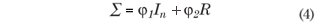where, φ1: nugget effect, φ2: sill value; R is a symmetric matrix that depends on φ3, R = R3) = [(riu)], in the order n x n with its elements of the diagonal rii = 1, i = 1, ..., n, where φ3 is function of the range (a) of the model and In is the identity matrix of the order n x n.

The parametric form of the covariance matrix, given in equation (4), applies for several isotropic processes, where the covariance C(si, su) is defined as C(hiu) = φ2riu, where hiu = ||sisu|| is the Euclidean distance between the points si and su. The variance of the stochastic process Z is C(0) = φ1 + φ2, and the semivariance can be defined as γ(h) = C(0)  C(h).

In many situations a data set with aberrant or discrepant observations can be considered influential, that is, they induce some type of decision in the construction of geostatistical models.

The local influence method proposed by Cook (1986) evaluates the simultaneous effect of observations on the maximum likelihood estimators without the need of its elimination from the data set. Christensen et al. (1993) studied diagnostic methods based on the elimination of cases in linear spatial models. The local influence technique has become known as a procedure to carry out sensitivity analyses of statistical models and has been widely used in linear models and nonlinear regression.

For an observed data set, let l(q) be the log-likelihood function of the proposed model, given in equation (3), where θ = (βT, φT)T, β = (β1,..., βp)T and φ = (φ1,..., φθ)T, and let w be a perturbation vector belonging to a space of perturbations Ω. Let l(θ/ω) be the log-likelihood function corresponding to the perturbated model for ω e Ω. It was assumed that there is ω0 e Ω such that l(θ) = l/ω0), for all θ and that l(θ/ω) is twice differentiable in (θT, ωT)T.

This study is justified by the importance of the modeling of spatial variability, since this process supplies parameters of spatial dependence structure that are used for the spatial interpolation of kriging.

Based on the interpolation of kriging, thematic maps are generated that could be used for a site-specific application of special inputs or site-specific soil management. The map quality depends on the quality of the inferences of the adjusted models. Therefore, to obtain trustworthy predictions that represent the real local variability by the interpolation produces, the modeling process must be very carefully carried out, mainly in the case of discrepant or influential observations.

The objective of this study was to use diagnostic techniques in Gaussian linear spatial models to evaluate the potential influence of atypical data on the parameter estimates that define the spatial dependence and to indicate the most robust models. For this purpose studies on local influence were conducted using the methods maximum likelihood and restricted maximum likelihood, to study the sensitivity of the models in the presence of influential observations.

MATERIAL AND METHODS

Influence of location

The following perturbation scheme was considered: Zω = Z + ω, with ω = (ω1, ..., ωn)Tvector of perturbation of the response and ω0 = (0,...,0)T, the point of no perturbation. The objective of this scheme of perturbation was to detect outliers in the data that affect the maximum likelihood estimator θ. Then, the perturbed log-likelihood function l(θ/ω), for the normal model is given byThe influence of the perturbation ω on the maximum likelihood estimator of θ can be evaluated by the likelihood displacement, defined by:where,is the maximum likelihood estimator of θ in the postulated model; and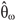is the maximum likelihood estimator of θ in the perturbed model.

Cook (1986) proposed to study the local behavior of LD(ω) around ω0, using the normal curvature Cl of LD(ω) in ω0 in the direction of a unit vector l and showed thatwith ||l|| = 1, where, L is the observed information matrix, evaluated in θ =; Δ is a (p + 3)x n matrix given by Δ = βT , ΔφT)T , evaluated in θ =and in ω = ω0, where, in this case:The information matrix L is defined as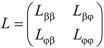, where,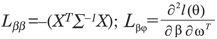, withand, with elements;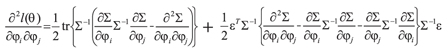.

Let Lmax be the eigenvector corresponding to the largest eigenvalue of B = ΔTL-1Δ. The graph of the elements of |Lmax| versus i (data order) can reveal which type of perturbation has the greatest influence on LD(ω), in the neighborhood of ω0 (Cook, 1986).

Simulation study

Simulation was carried out by Monte Carlo experiments, where simulated data sets were arranged in a regular grid (10 m distance between points), totalizing 100 points, with known structures of spatial dependence, by means of Gaussian stochastic processes. The simulation of stationary spatial processes of second order was carried out by the Cholesky decomposition method, a matrix operation which, applied to the vector of random numbers generated produces another vector with random numbers with the characteristic of a given correlation matrix between them (Johnson & Wichern, 1982).

The covariance structures of the models used in the simulations were exponential, Gaussian and Matérn, with κ = 0.7 and 3.0. In all cases, a constant mean of µ = β = 9.45 was considered. Four parameter vectors were used for each model φ = (φ1, φ2, φ3)T : 1st case: φ = (0, 10, 10)T; 2nd case: φ = (0, 10, 15)T; 3rd case: φ = (0, 10, 20)T; 4th case: φ = (0, 10, 60)T.

The perturbation scheme used had been proposed by Ortega et al. (2002), as presented in the equation (8) below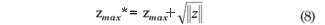where, zmax* is the new value maximum of the vector and zmax is the maximum value of vector z;. Consequently, the perturbation vector ω can be expressed as : ω = (0, ...,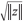, ..., 0)T.

The structure of spatial dependence was modeled for the simulated data sets, but adding the perturbation vector ω. Diagnostic techniques were applied to evaluate the sensitivity of the models to the perturbation scheme.

The methods of maximum likelihood and restricted maximum likelihood were used for the parameter estimation and in the diagnostic analyses (Mardia & Marshall, 1984 and Christensen et al. 1993). Because the results were very similar, only the graphs based on maximum likelihood are presented.

Experimental study

The data of the soil chemical properties were collected in an area of commercial grain production of 71 ha, in the 2006/2007 growing season, in the county of Cascavel, Western region of Paraná, (lat 24.95 º S, long 53.57 º W, average altitude 650 m asl. The soil was classified as dystroferric Red Latosol and the climate of the region is Temperate mesothermal and superhumid, climate type Cfa (Köppen), with an average annual temperature of 21 ºC.

A centered systematic sampling with pairs of adjacent points was carried out (lattice plus close pairs), with a maximum distance of 141 m between points. At some places the sampling was carried out at distances of 75 and 50 m between points. All samples were geo-referenced with a GPS (Global Positioning System) signal receiver.

In the vicinity of a few points four soil sub-samples were randomly collected, from the layer 0.00.2 m. The sub-samples of approximately 500 g were mixed and stored in plastic bags, to compose a representative sample of the plot.

Initially, an exploratory statistical analysis of the data was carried out to evaluate the general behavior and to identify to the presence of discrepant points and their possible causes. Among the analyzed chemical properties, discrepant points were observed for soil P. This trait was analyzed in this study.

Thereafter, a spatial data analysis was performed using geostatistical techniques, identifying the structure of spatial dependence by means of the adjustment of some theoretical models, with parameters estimated by maximum likelihood (ML) and restricted maximum likelihood (RML). In this stage, the criteria of model validation and diagnostic techniques were applied for the posterior construction of maps of the study variable. All analyses were carried out using software R (Ihaka & Gentleman, 1996) and the modules: geoR (Ribeiro Júnior & Diggle, 2001) and Splancs (Rowlingson & Diggle, 1993).

RESULTS AND DISCUSSION

Local influence on simulated data

Estimates of maximum likelihood (ML) and restricted maximum likelihood (RML) for 1, j2and j3 , using the exponential, Gaussian and Matérn covariance functions, are presented in table 1.

The results of the exponential model 0-10-10 (Table 1) show that the methods ML and RML overestimated the parameters φ1 and φ3 and underestimated parameter φ2. A similar behavior was identified in the parameter estimates of the exponential model 0-10-60, except for parameter φ3 which had been underestimated when it was estimated by MV. The variables simulated by the Gaussian 0-10-10 and Gaussian 0-10-15 model overestimated the parameters φ1 and φ3 and underestimated parameter φ2 by the methods of ML and RML. By the Matérn model 0-10-15 ê = 0.7, the estimated φ1 values were equal to those supplied in the simulation and the φ3 values were close to the simulated. However, the values of parameter φ2 were overestimated. By the Matérn model 0-10-10, k = 3.0, it was observed that φ2 was clearly overestimated. Hence, the perturbed values in the simulated variables with exponential, Gaussian and Matérn (k = 0.7 and 3.0) covariance functions, had a rather strong influence on parameter estimation, by ML as well as by RML.

Figures 1 to 8 present the graphs of diagnostic techniques to identify perturbed observations. The results of the local influence analysis showed that the index plots of |Lmax| highlighted the perturbed observations for all variables, in the parameter estimation by ML as much as RML (not shown here).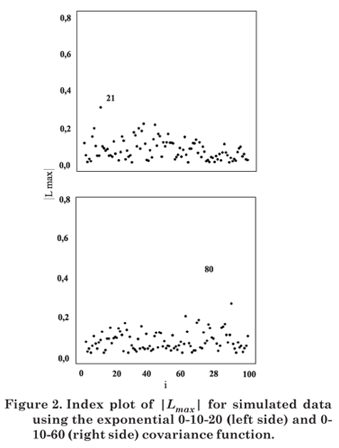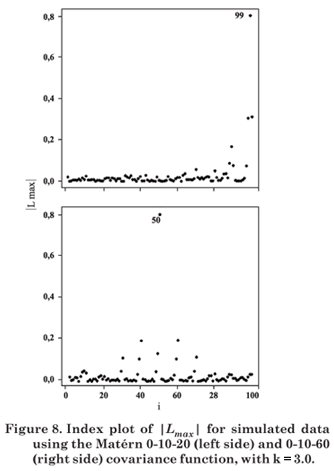Hence, in this simulation study diagnostic measures were effective to detect all perturbed observations.

Spatial analysis of influence of discrepant points on phosphorus content

After sampling, soil samples were sent to the laboratory for chemical analyses. In the first analysis, the P content presented three discrepant values (60.0; 38.6 and 60.0 mg dm-3) in the plots 1, 26 and 45, respectively (Figure 10a). The soil chemical analysis was repeated in these plots and no changes in the values were observed. The data of 1, 26 and 45 are therefore not measurement or laboratory errors; they represent real values of the local soil conditions.

Graphical diagnostic techniques were applied to evaluate whether the discrepant points 1, 26 and 45 or others exert some type of influence on likelihood displacement, on the covariance function and the linear predictor. The index plots of |Lmax| were used to evaluate the influence.

Diagnostic graphs are presented in figure 9 for the exponential, Gaussian and Matérn (κ = 0.7 and 3.0) covariance functions, by ML. The index plots of |Lmax| indicated the observations 1, 26 and 45 as potentially influential.

Influence on descriptive analyses

A descriptive summary of the phosphate content was presented (Table 2) with all collected data and without the discrepant observations. It was verified that the mean P content (15.80 mg dm-3) is well above the recommended upper limit (EMBRAPA, 1979). Furthermore, the data were highly heterogeneous, since the variation coefficient was very high (CV = 72.76 %), due to the discrepant values of the observations 1, 26 and 45, identified for the Box-plot (Figure 10b).

Since the statistical techniques applied in this paper assume that the data have normal distribution, the Box-Cox was transformed with lambda = -0.7. Also, the descriptive analyses are presented for the data set without the observations 1, 26 and 45 (P-1-26-45), verifying the influence in the descriptive analysis. Differences were observed between the means of the variable with all data or when removing influential observations. The same behavior was observed in the standard deviations. The analysis of the coefficient of variation (CV) showed that without the observations 1, 26 and 45 the CV value was much lower than with all data. However, as the CV is > 30 %, the data were still considered heterogeneous (Gomes, 2000).

Influence on the parameter estimates

The results of the analyses of spatial variability are presented for the original data and for the data set without the observations 1, 26 and 45 (Table 3). The results of the estimation of the parameters β, φ1, φ2 and φ3, were presented for the exponential, Gaussian and Matérn covariance functions, using ML and RML. The values in brackets stand for the standard deviations of the estimated parameters. Overall, the values of the estimators of β, φ1, φ2 without the influential observations were lower than when estimated with the original data. The estimates ML of φ3, without the influential observations were greater than those obtained with the original data. This was not the case for parameter φ3, when estimated by RML.

The cross-validation criteria (Faraco et al., 2008) applied to the models in the study, both with all observations and without the observations 1, 26 and 45 indicated the Gaussian model using RML as best fitting.

Influence on the construction of thematic maps

Figure 11 presents the thematic maps for P content with original data and without the observations 1, 26 and 45 based on the interpolation by ordinary kriging. The maps had been constructed using the models indicated for the cross-validation criteria. The variation in the color scale between the maps was considerable. The map for the original data set (Figure 11a) shows that the area comprises regions with a P content of > 18 mg dm-3 (Embrapa, 1997). In the map, constructed without the influential observations considered (Figure 11b) no region has values > 18 mg dm-3. This indicates that observations 1, 26 and 45 also exert a strong influence on the construction of the thematic maps.

Thus, if the construction of the thematic maps does not take the diagnostic analyses into consideration, which detects outliers, the distribution map of the P content for producers would overestimate P concentrations in the study area. Consequently, the principles of the precision agriculture would be disregarded, since the soil correction would not be locally adjusted.

The results showed that the removal of the influential data led to an increase of 10.14 % of the area of the first class; an increase of 17.16 % of the second and a decrease of 7.03 % of the area of the third class in the map (Table 4). However, the fourth and fifth class were not identified in the map when the influential data had been removed.

CONCLUSIONS

The study with simulated data showed that the proposed diagnostic techniques were able to identify the perturbed data. The restricted maximum likelihood estimator produced unbiased estimates of the parameters of spatial dependence. For the results obtained with real data, the study concluded that the presence of atypical cases in the data had a strong influence on the thematic maps, due to change in the structure of the spatial dependence. The use of diagnostic techniques should be part of all geostatistical analyses, to ensure the high quality of the information contained in the thematic maps. The elimination of atypical cases can produce maps that are inappropriate.

ACKNOWLEDGEMENTS

The authors gratefully acknowledge the financial support provided of the Brazilian Federal Agency for Support and Evaluation of Graduate Education (CAPES), National Council of Scientific and Technological Development (CNPq), National Supply Company (CONAB) and Fundação Araucária, Brazil and Project Dipuv 11/2006, Universidad de Valparaíso, Chile.

LITERATURE CITED

Recebido para publicação em fevereiro de 2008 e aprovado em agosto de 2009.

• CHRISTENSEN, R.; JOHNSON, W. & PEARSON, L. Covariance function diagnostics for spatial linear models. Inter. Assoc. Mathem. Geol., 25:145-160, 1993.
• COOK, R.D. Assessment of local influence (with discussion). J. Royal Statis. Soc., Series B, 48:133-169, 1986.
• CRESSIE, N.A.C. Statistics for spatial data. New York, John Wiley & Sons, 1993. 900p.
• EMPRESA BRASILEIRA DE PESQUISA AGROPECUÁRIA - EMBRAPA. Serviço Nacional de Levantamento e Conservação de Solos. Manual de métodos de análise de solo. Rio de Janeiro, Ministério da Agricultura, 1979. 247p.
• EMPRESA BRASILEIRA DE PESQUISA AGROPECUÁRIA - EMBRAPA. Serviço Nacional de Levantamento e Conservação de Solos. Manual de métodos de análise do solo. 2.ed. Rio de Janeiro, Centro Nacional de Pesquisas de Solos, 1997. 212p.
• FARACO M.A.; URIBE-OPAZO, M.A.; SILVA, E.A.; JOHANN J.A. & BORSSOI, J.A. Seleção de modelos de variabilidade espacial para elaboração de mapas temáticos de atributos físicos do solo e produtividade da soja. R. Bras. Ci. Solo, 32:463-476, 2008.
• GOMES, P. Curso de estatística experimental. 14.ed. Piracicaba, Degaspari, 2000. 477p.
• IHAKA, R. & GENTLEMAN, R. A language for data analysis and graphics. J. Comput. Graphical Statistics, 5:229-314, 1996.
• JOHNSON, R.A. & WICHERN, D.W. Applied multivariate statistical analysis. Madison, Prentice Hall International, 1982. 607p.
• MARDIA, K. & MARSHALL, R. Maximum likelihood estimation of models for residual covariance in spatial regression. Biometrika, 71:135-146, 1984.
• ORTEGA, E.; BOLFARINE, H. & PAULA, G. Influence diagnostics in generalized log-gamma regression models. Comput. Statistics Data Anal. J., 42:165-186, 2002.
• RIBEIRO JUNIOR, P.J. & DIGGLE, P.J. geoR: A package from geoestatistical analysis. R. News, 1:15-18, 2001.
• ROWLINGSON, B. & DIGGLE, P.J. Splancs: Spatial point pattern analysis code in S-Plus. Comp. Geosci., 19:627-655, 1993.
• (1
) Parte da Dissertação de Mestrado do primeiro autor.

# Publication Dates

• Publication in this collection
09 Feb 2010
• Date of issue
Dec 2009

• Accepted
Aug 2009